# Neural Networks: Everything you Wanted to KnowNeural Networks: Everything you Wanted to Know

## Introduction

The goal of this post is to:

• Make a brief introduction on what are Neural Networks and how they work
• Make a detailed mathematical explanation of how they learn

## Neural Networks

In a general sense, neural networks are a method to approximate functions. Following the universal approximation theorem:

A neural network with a single hidden layer is sufficient to represent any function in a given range of values, although it is possible that the hidden layer is so large that it makes implementation impossible or that it is not possible to find the appropriate weights.

If we consider that normally any problem you can think of can be decomposed and modeled in the form of mathematical functions, according to this theorem, neural networks should be able to solve any problem, right?

There are two major limitations of neural networks:

• They are only able to approximate continuous functions
• And within a certain range of values

Roughly speaking, with a neural network we can detect patterns and use them to solve problems. Furthermore, with deep neural networks we can transform the data space until we find a representation that facilitates the achievement of the desired task.

They would perform something similar to what is represented in the image below: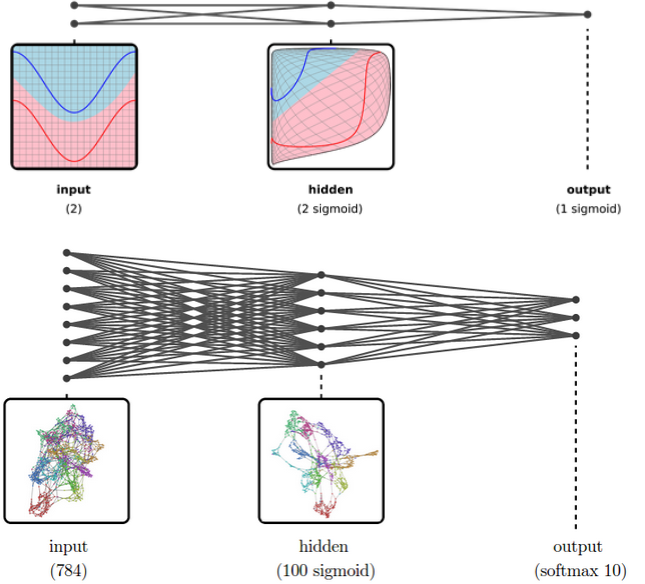Basically, they transform input data into other spatial representation to be able to differentiate it in an easier way.

The following example is really representative:

If we were trying to solve the classification problem of the following dataset with a linear classificator, it would be really difficult, as the data points are not linearly separable.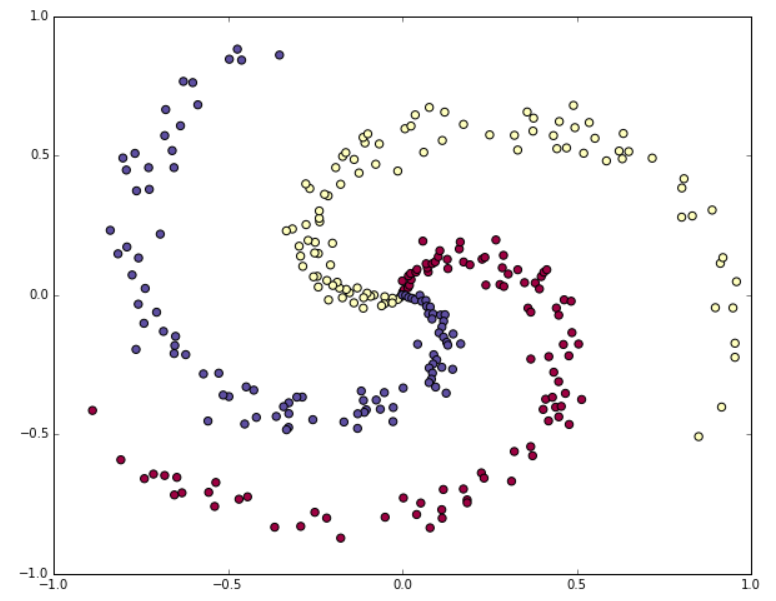We would obtain something similar to: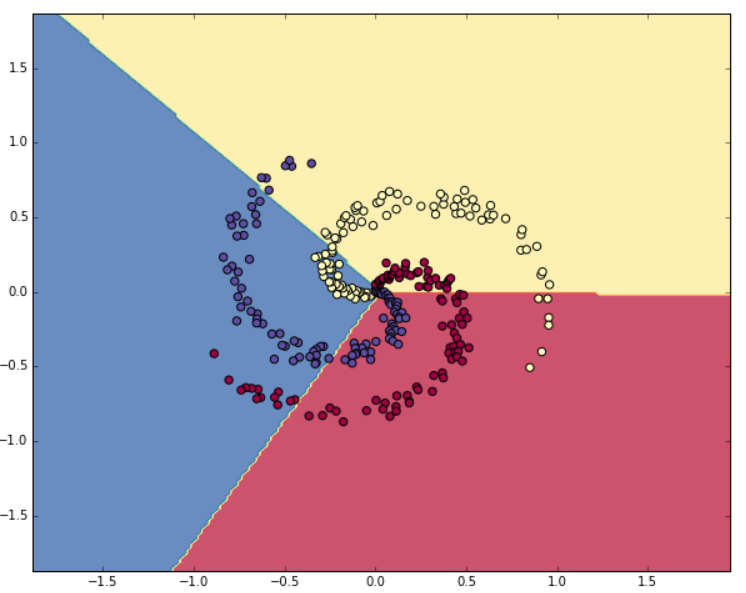But as we can see, it is not quite good. Do you want to see what a neural network is capable of?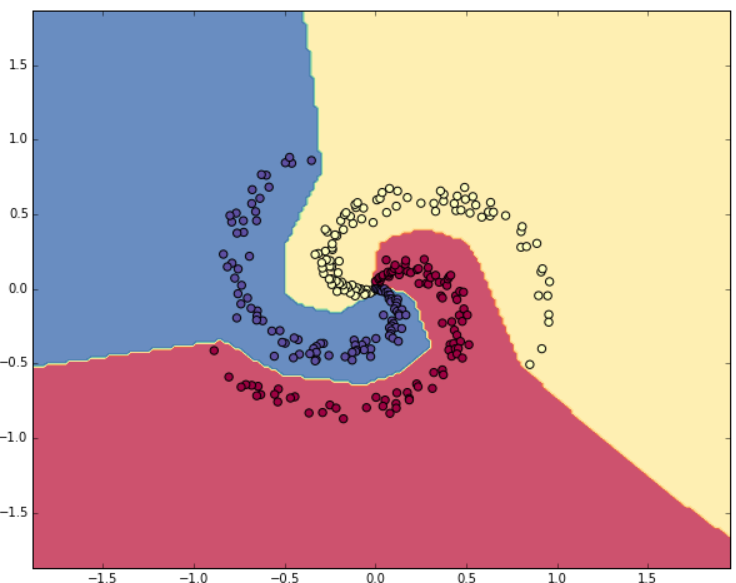This looks way better ;)

## What Neural Networks are Not Able to Do

Neural Networks cannot give exact solutions to a problem. For example, a neural network would have a really hard time implementing a simple multiplication.

• First, because we would demand exact values from it.
• And second, because as we said before, they are capable of approximating functions in a given range. Multiplication would require a range of [-inf, +inf].

Furthermore, they cannot “think” either. They are just very powerful pattern detectors that give the sensation of intelligence, but they don’t have it. We have to provide the intelligence ourselves.

We also have to take into account that although they are very useful because they solve problems that up to now have been very complex for a computer, such as detecting different types of dog breeds or traffic signs, it is very difficult to extract that knowledge from them.

In other words, they are capable of doing what we ask them to do, but it is difficult to find out how exactly they are doing it.

# How do Neural Networks Learn?

Basically, Neural Networks learning process is similar than human learning. They learn by repetition.

They see something, make a guess of what is it, and other agent tells them if they are right or not. If they are wrong, they will adjust their knowledge to avoid repeating the same mistake.

## Let’s see a concrete example

As you may know, neural networks are made up of layers, which in turn are made up neurons.

In this link, you can see the complete example: https://mattmazur.com/2015/03/17/a-step-by-step-backpropagation-example/

In the image below we can see a feed-forward type of network, with an iput layer of 2 neurons, a hidden layer of 2 neurons and an output layer of other 2 neurons. Aditionally, the hidden and output layers have a bias too.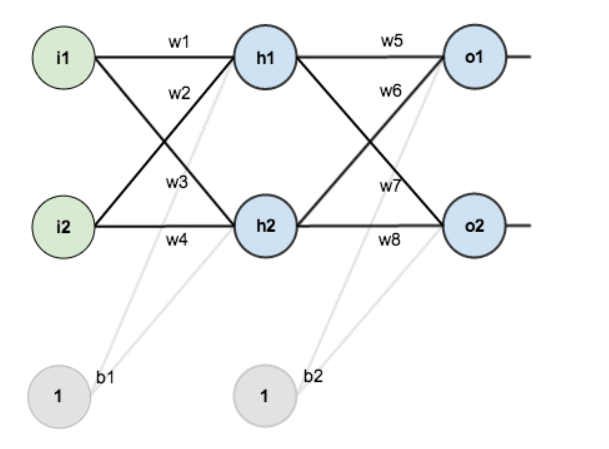There are many more architectures, but this is the most used one. In this type of architecture, each neuron of the i-layer connects with all the neurons of the i+1 layer.

That is, neurons in one layer are only allowed to connect to neurons in the next layer. These types of layers are known as dense or fully connected layers.

For simplicity, let’s assume that our training set is composed of only 1 element: 0.05, 0.1, which is class 1 (it could be class 0, if the probability of neuron o1 is greater than that of neuron o2, or class 1 if the opposite occurs).

In the following image you can see the network with the randomly initialized weights, the training set element at the input, and the desired output: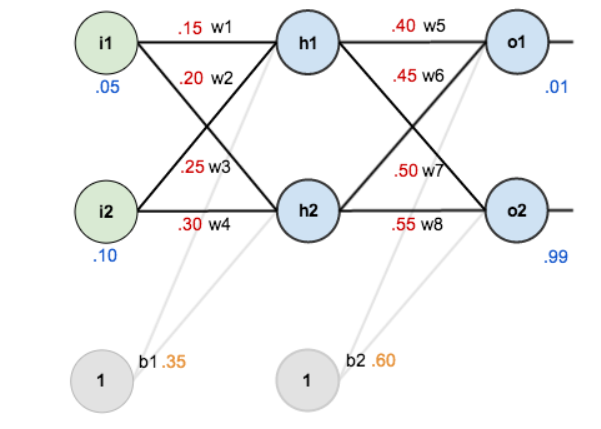Once our Neural Network is defined and ready to be trained, let’s focus on how they learn.

The 2 most common learning methods are:

• Forward pass
• Back propagation

## Forward Pass

It consists of calculating the output of the network with the current weight values. To do so, we feed the network with our training element.

But, first of all, let’s see how a neuron really is: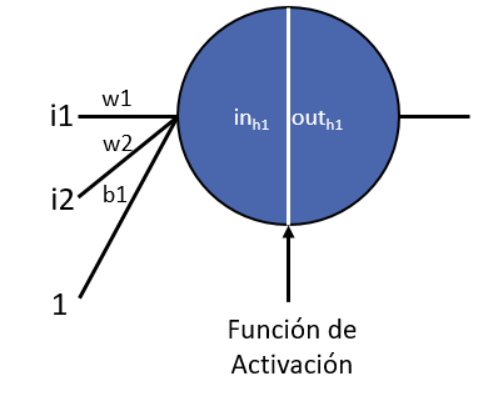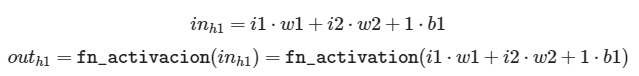Where fn_activation is the activaction function chosen. Some of the most popular activation functions are: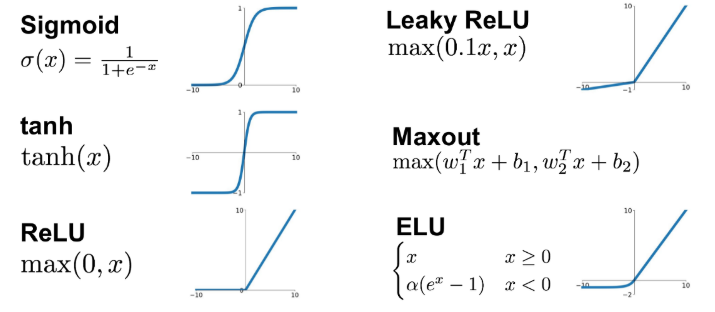https://towardsdatascience.com/complete-guide-of-activation-functions-34076e95d044

## Are Artificial Neural Networks Similar to Real Neural Networks?

Artificial Neural Networks are inspired in the the human brain. In fact, we have more or less 10 billion neurons, each of the interconnected with other 10.000 neurons.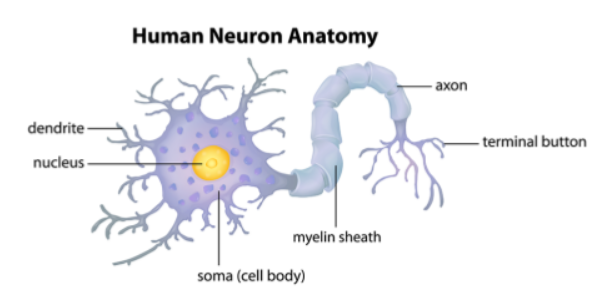https://training.seer.cancer.gov/anatomy/nervous/tissue.html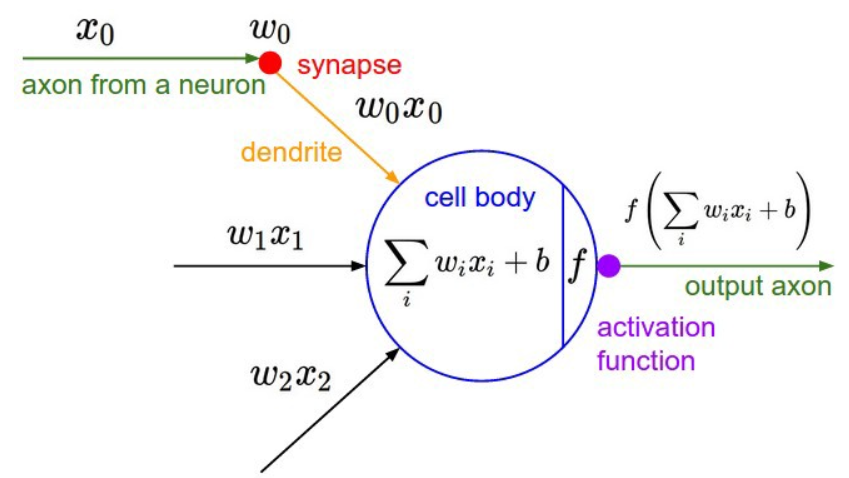https://mattmazur.com/2015/03/17/a-step-by-step-backpropagation-example/

The body of the neuron is called the soma, the entrances, dendrites, and the exits, axons. The magic of the brain is in the thousands of connections of some dendrites with the axons of other neurons.

The way it works is that each neuron receives an electrochemical impulse from other neurons through its axons (outputs) and the dendrites (inputs) of the receptor.

If these impulses are strong enough to activate the neuron, then this neuron passes the impulse on to its connections. By doing this, each of the connected neurons checks again if the impulse that reaches the soma from the dendrites (inh1 in our previous example) is strong enough to activate the neuron (fn_activation is in charge of checking this) and expand by more neurons.

Taking into account this mode of operation, we realize that the neurons are really like a switch: either they pass the message or they don’t.

Neural networks are not based on their biological partners, but are inspired by them.

## Forward & Back Propagation

Now, we will understand how the network calculates the output outh1.

Taking a look at the network again: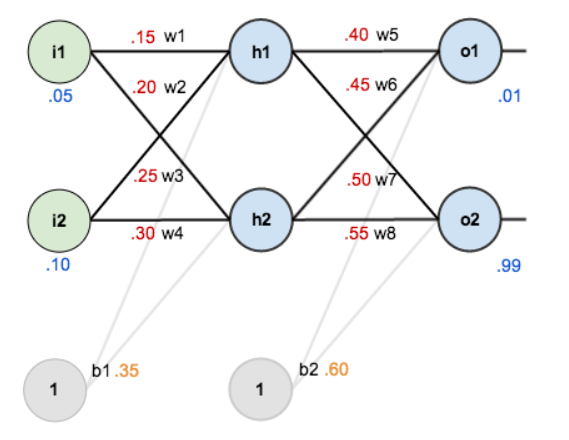https://mattmazur.com/2015/03/17/a-step-by-step-backpropagation-example/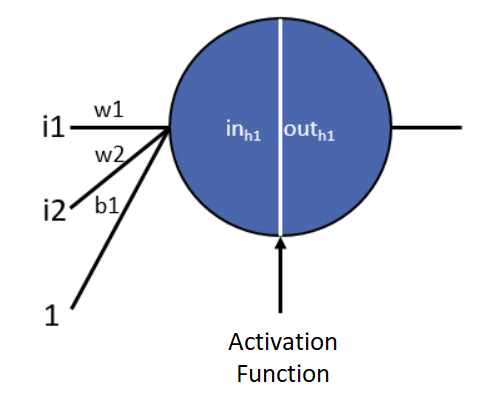https://mattmazur.com/2015/03/17/a-step-by-step-backpropagation-example/

IMAGE

To calculate o1 and o2 we need:

For the first layer, the hidden layer: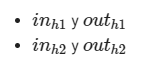For the last layer, the output one: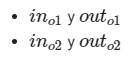Where: o1 = outo1 and o2 = outo2.

So we need to calculate: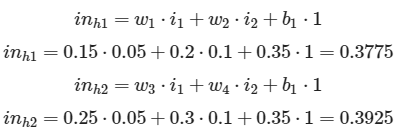Now, to obtain outh1 and outh2, we need to apply the fn_activation. In this case we have selected the logistic functionas the activation one: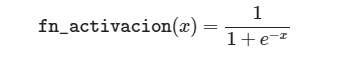so: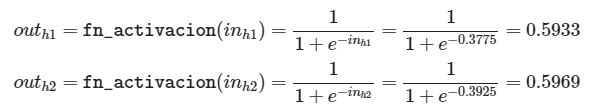Let’s see how we are in the output calcualation process: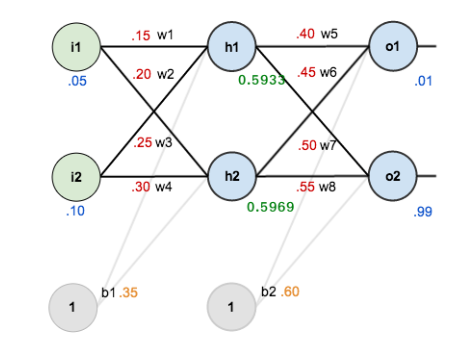https://mattmazur.com/2015/03/17/a-step-by-step-backpropagation-example/

We just need to calculate now o1 and o2: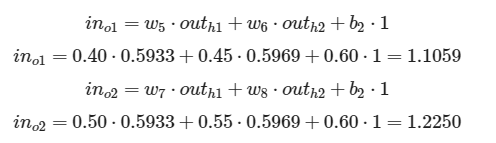So, applying the activation function: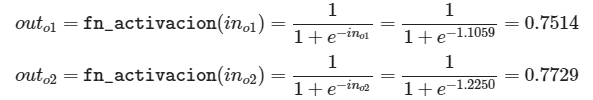Finally! We have our neural network predictions calculated! But hold on, our predictions are terrible! They’re nothing like the 0.01 and 0.99 desired! How do we fix this?

How about we calculate the total error and try to minimize it? In fact, that’s precisely what the backpropagation algorithm does: it updates the weights according to how much each weight influences the total error in order to minimize it

Let’s calculate the total error: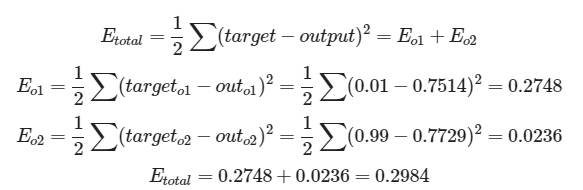Notice how the outo2 error is much smaller than the outo1 error. This is because 0.7729 is much closer to 0.99 than 0.7514 of 0.01, so the change should be greater in the neurons involved in the calculation of outo1 than in outo2 .

And how can we update the weights, each according to what influences the total error? It’s simple, just by calculating how much a change in a given weight influences the total error, and updating it in line with this relationship.

For example, can you think of a way to calculate how much the weight w5 influences the total error? In other words, how much does a change in w5 weight influence the total error? Doesn’t this sound familiar?

We’re talking about derivatives! Look, we can understand each neuron as a function, and apply the rule of the chain to get from the total error to the w5 weight. But first, do you remember how the chain rule works?

Let’s see an example: Imagine that we want to derivate the function: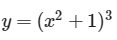In other words, we want to find out how much a change in the x variable affects the y variable.

We can understand this function as a composition of other 2, where: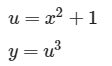Now, we need to derivate y in respecto to x. To do so, we need to derivate first y in respect to u, and then, u in respect to x.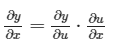In our example: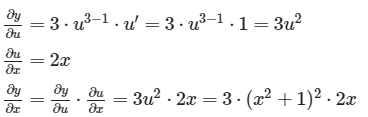This was the chain rule applied. Let’s see it now as it were a graph: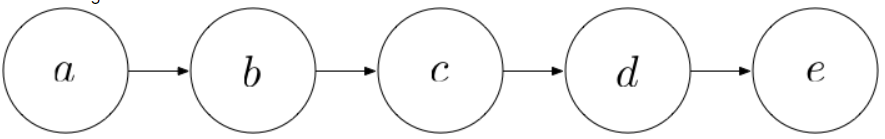Imagine that every circle is a function and every arrow a multiplication. Then, using the chain rule we could write:Now, let’s see how we can write the formula that calculates how the Etotal changes with respect to the weight w5.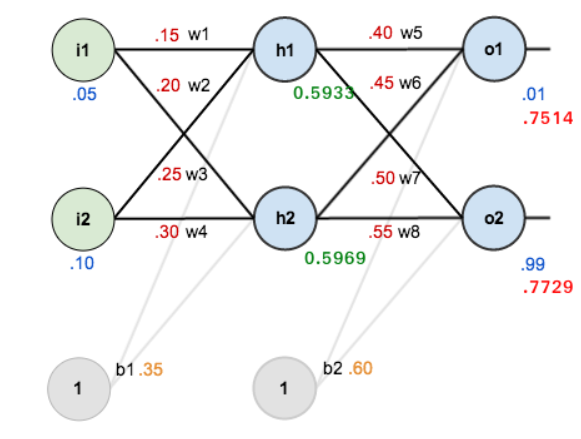https://mattmazur.com/2015/03/17/a-step-by-step-backpropagation-example/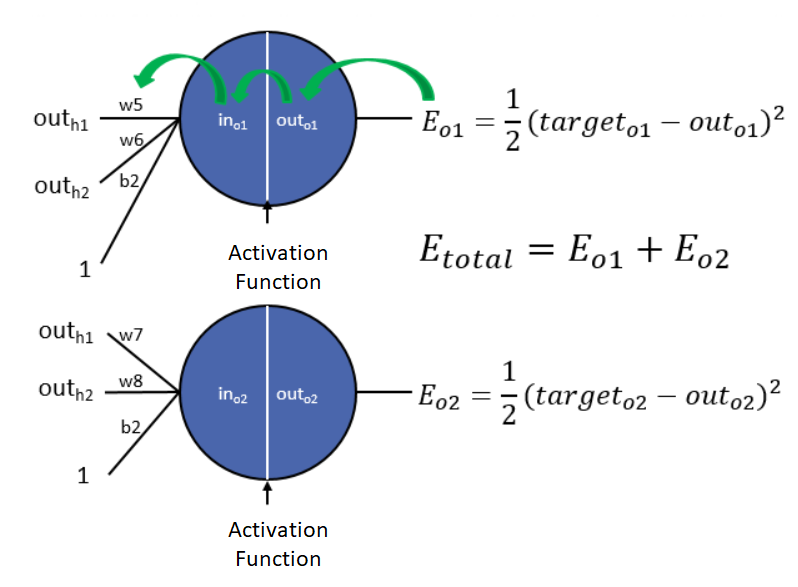https://mattmazur.com/2015/03/17/a-step-by-step-backpropagation-example/

As Jack the Ripper said: lets go for parts. The total error is defined as: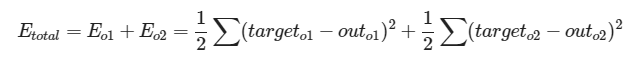As we can see w5 just affects the o1 neuron, so we just care about the Eo1 which makes our Etotal = Eo1.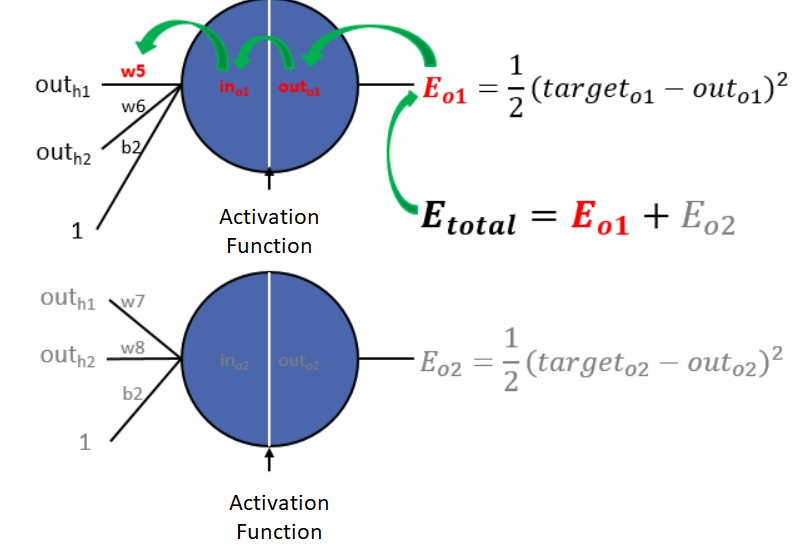https://mattmazur.com/2015/03/17/a-step-by-step-backpropagation-example/

So we could define the variance of the w5 weight with resepct to Etotal as: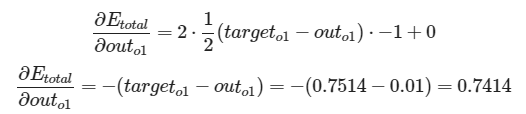Great! Let’s go with the next term: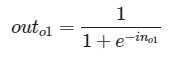That can be expressed as: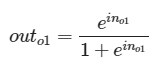And its derivative: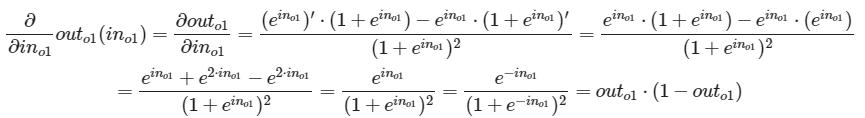The last two steps are possible because the derivative of the logistic function is an even function, i.e. f(x)=f(-x) .

All right, let’s get back to business. We already have the derivative so now we have to calculate its value: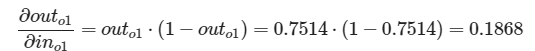WE already have the first and second terms and we just need to calculate the highlighted term to get to w5: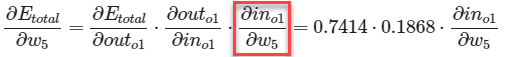If we recall, in01 was: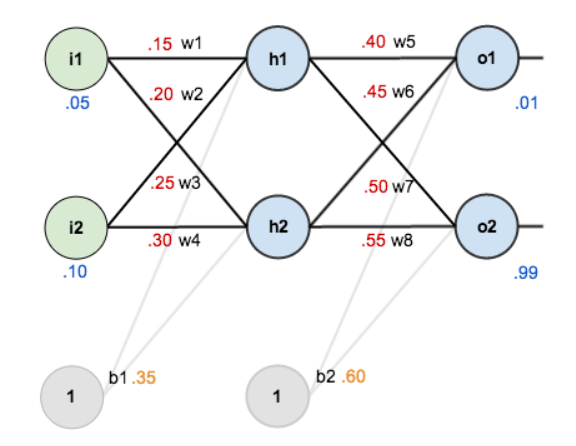https://mattmazur.com/2015/03/17/a-step-by-step-backpropagation-example/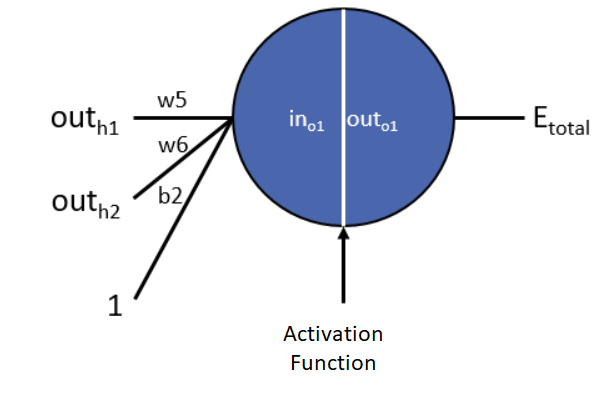https://mattmazur.com/2015/03/17/a-step-by-step-backpropagation-example/

And if we take a look at the inputs of the o1 neuron we could calculate inmediately the in01 formula: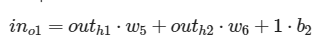And now, we are able to calculate our term;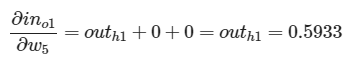Finally! We already have all the necessary terms to know how much w5 affects Etotal: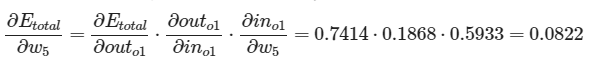We already know how to calculate how much the weights between the second layer and the terera (the hidden and the exit) influence. But… how do we find out what the weights between the first layer and the second layer (the entrance and the exit) influence?

Very simple, in the same way as we did before!

What is the first thing we need to define?

The derivative of Etotal with respect to the weight w1. And how is that defined?

Here is the network: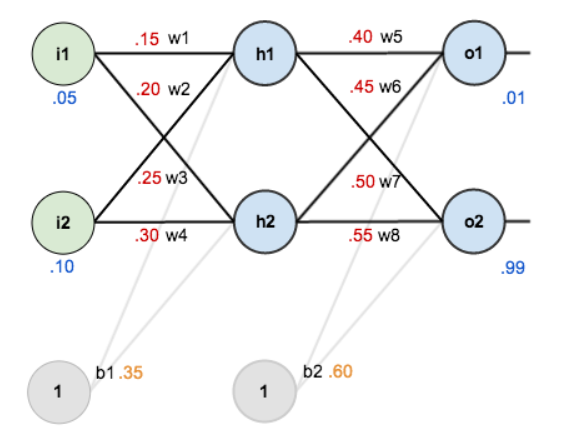https://mattmazur.com/2015/03/17/a-step-by-step-backpropagation-example/

Let’s deconstruct the neural network in its neurons: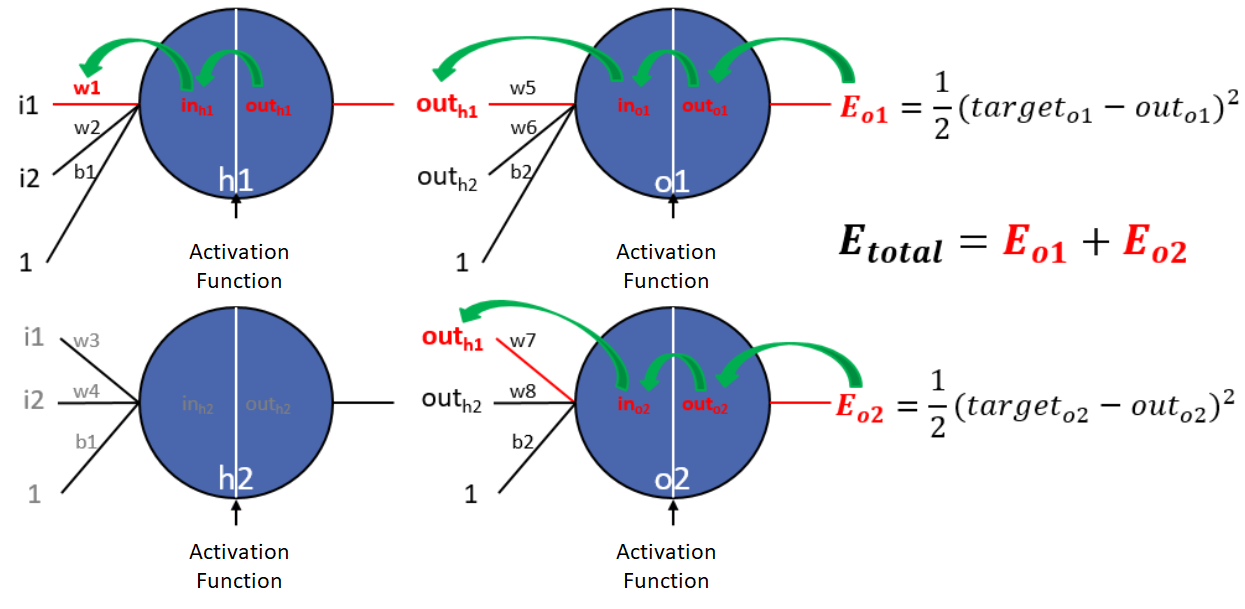https://mattmazur.com/2015/03/17/a-step-by-step-backpropagation-example/

Now, we have 2 possible paths to follow: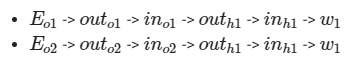So, we need to calculate the errors corresponding to the 1 and 2 neurons: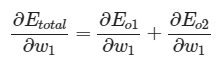This means that we now have two sources of error affecting weight w1 , which are Eo1 and Eo2 . How is each defined? Very easy, what is the first element that we would find if we were to walk from Eo1 to weight w1 in the network diagram? outo1 , right?

With which, we already have to: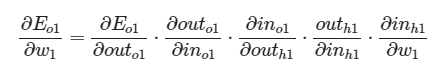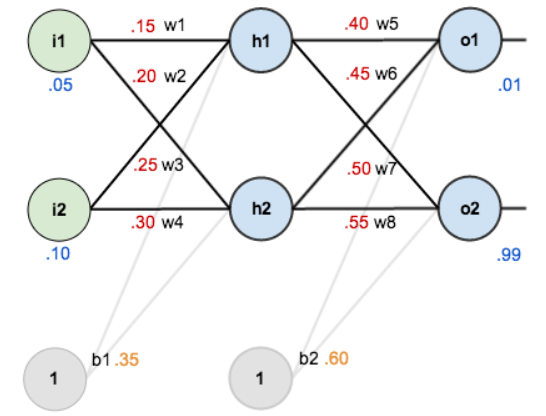https://mattmazur.com/2015/03/17/a-step-by-step-backpropagation-example/

Let’s solve to Eo1: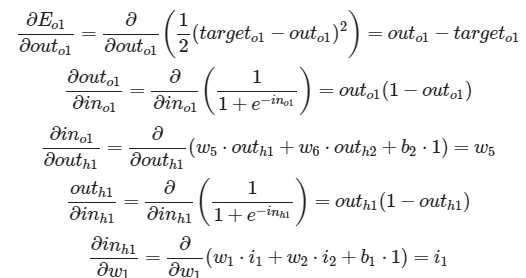Now we have how much varies Eo1 with respect to w1. But we are lacking something, if we take a look at the neural network again: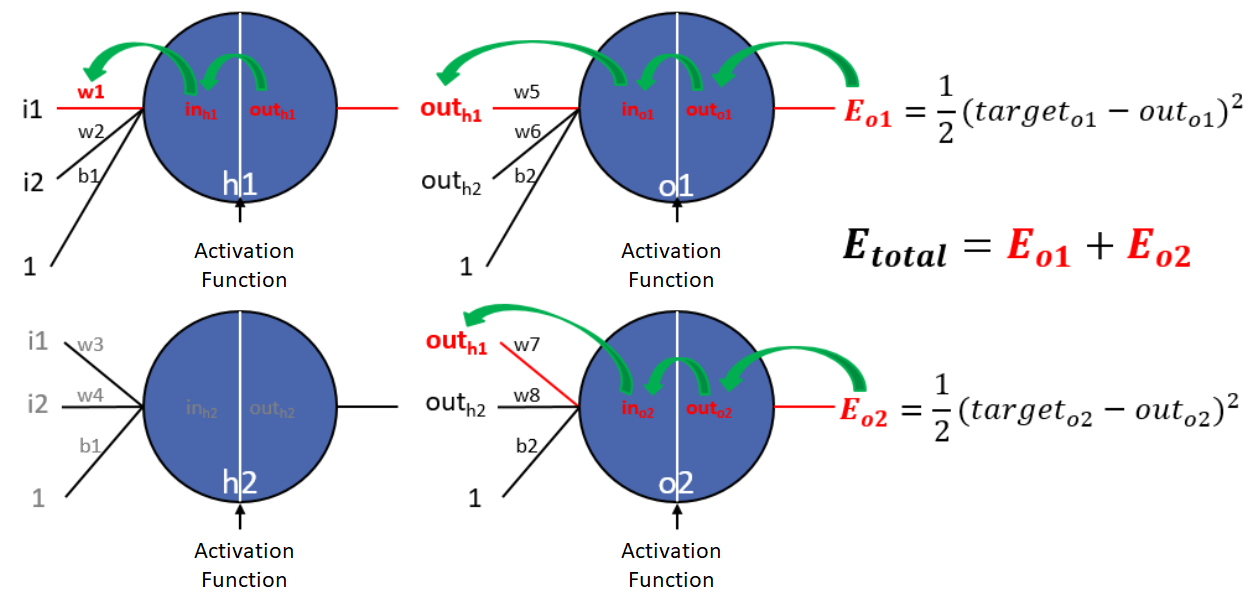https://mattmazur.com/2015/03/17/a-step-by-step-backpropagation-example/

We also need the error corresponding to the second neuron, Eo2 . With this error, we could calculate how much the weight w1 influences the Etotal, which is what we are interested in: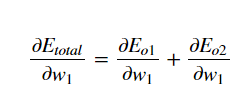And repeating this for each weight, we would have everything necessary to apply the descent of the gradient on our weights, and thus update them, see: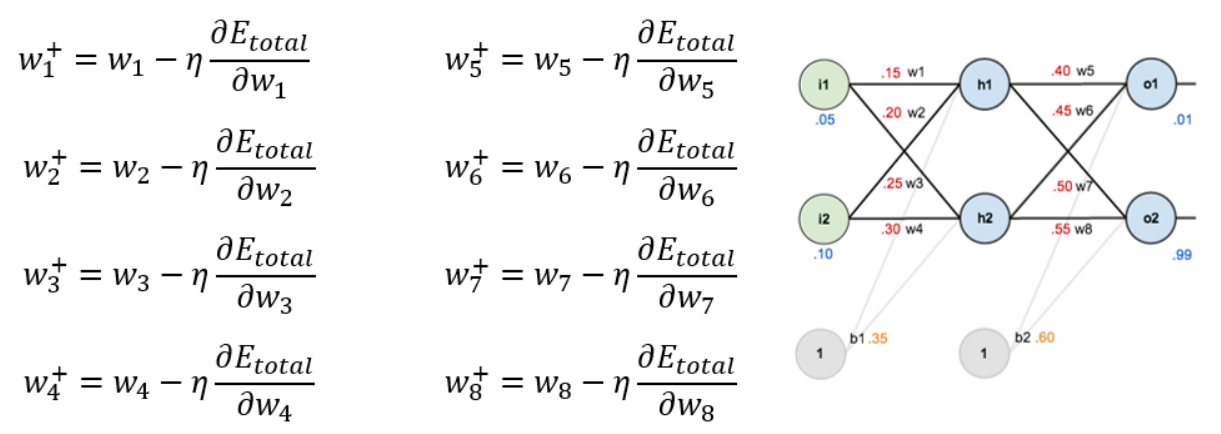https://mattmazur.com/2015/03/17/a-step-by-step-backpropagation-example/

Where η is the learning rate, which indicates how big a step we want to take in the opposite direction of the gradient.

## Final Words

As always, I hope youenjoyed the post, that you have learned about neural networks and how do they use mathematics to learn themselves!